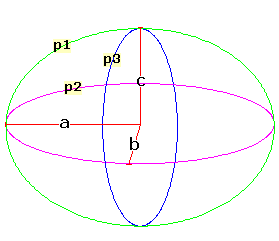Spike's Calculators

# Ellipsoid

Calculate the volume and the surface area of an ellipsoid.This calculator works for any measurement unit. For example, if you calculate inches, inches will have to be used in all the length fields, and the result of the areas will be square inches; the volume results in cubic inches and length measurements in the starting unit, inches.

### Ellipsoid

Length a #
Length b #
Length c #
Decimal Precision #

#### Results:

 Ellipsoid Volume #³ Surface Area #² Circumference - p1 # Circumference - p2 # Circumference - p3 # Surface to Volume Ratio sa:vol

#### Calculation

• length a
• length b
• length c
• decimal precision, number of digits after the decimal point

#### Results

• the volume of the ellipsoid (unit cubed)
• the surface area of the ellipsoid (unit squared)
• circumference of p1 using a and c (unit)
• circumference of p2 using a and b (unit)
• circumference of p3 using c and b (unit)
• surface area to volume ratio (decimal)
##### Formula
```V = 4/3Πabc
SA = 4/3Π((ab1.6 +ac1.6+bc1.6 )/3)1/1.6
h = (a-c)²/(a+c)²
C = Π(a+c)(1+(3h/(10+√ 4-3h));
s/v = SA/V
where V is the volume of the ellipsoid
SA the surface area
C the circumference of an ellipse
s/v the surface area to volume ratio
Π = 3.14159265
a , b, and c the axis lengths
```